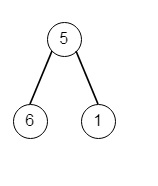# Maximum Average Subtree in Pythonn

Suppose we have the root of a binary tree; we have to find the maximum average value of any subtree of that tree. So if the tree is like −The output will be 6, this is because, for node 5, it will be (5 + 6 + 1)/ 3 = 4, then for node 6 it will be 6 / 1 = 6, and for node 1, it will be 1 / 1 = 1, so the max is 6.

To solve this, we will follow these steps −

• res := 0

• Define a method called solve(), this will take root

• if root is not set, then return a pair [0, 0]

• left := solve(left of root) right := solve(right of root)

• c := left + right + 1

• s := left + right + value of root

• ans := max of and ans s/c

• return a pair[c, s]

• From the main method, set ans := 0, call solve(root) and return ans

## Example(Python)

Let us see the following implementation to get better understanding −

Live Demo

class TreeNode:
def __init__(self, data, left = None, right = None):
self.data = data
self.left = left
self.right = right
def insert(temp,data):
que = []
que.append(temp)
while (len(que)):
temp = que
que.pop(0)
if (not temp.left):
if data is not None:
temp.left = TreeNode(data)
else:
temp.left = TreeNode(0)
break
else:
que.append(temp.left)
if (not temp.right):
if data is not None:
temp.right = TreeNode(data)
else:
temp.right = TreeNode(0)
break
else:
que.append(temp.right)
def make_tree(elements):
Tree = TreeNode(elements)
for element in elements[1:]:
insert(Tree, element)
return Tree
class Solution(object):
def helper(self, node):
if not node:
return 0, 0
left_sum, left_count = self.helper(node.left)
right_sum, right_count = self.helper(node.right)
self.ans = max(self.ans, (left_sum + right_sum + node.data) / (left_count + right_count + 1))
return left_sum + right_sum + node.data, left_count + right_count + 1
def maximumAverageSubtree(self, root):
self.ans = 0
self.helper(root)
return self.ans
ob = Solution()
root = make_tree([5,6,1])
print(ob.maximumAverageSubtree(root))

## Input

[5,6,1]

## Output

6.0